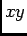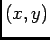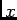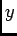# OOF: Finite Element Analysis of Microstructures

/output/cross_section/nonuniform/functionNext: The Element Types Up: /output/cross_section Previous: /output/cross_section/uniform/function   Contents

Subsections

## /output/cross_section/nonuniform/function

Plot the given function along a given line in theplane, using nonuniformly spaced plot points. The points will be placed within the specified tolerance of the element boundaries.

### cross_section/nonuniform arguments

filename
Where the data will go.
start
Thecoordinates of the beginning of the line along which the data will be plotted. The coordinates must be given as a comma-separated pair in parentheses, e.g, (1.2, 3.4). The coordinates are in the coordinate system of the screen, so to plot values relative to the coordinate system of the undistorted mesh, make sure to set /graphics/enhancement=0 (see sections 3.9.3 and 5.2.6).
finish
Thecoordinates of the end of the line.
tolerance
The minimum distance between plotted points.
x
Should thecoordinate of the plotted points be printed?
y
Should thecoordinate of the plotted points be printed?
s
Should the arclength coordinate of the plotted points be printed? The arclength is the distance from the starting point.Next: The Element Types Up: /output/cross_section Previous: /output/cross_section/uniform/function   Contents
/* Send mail to the OOF Team *//* Go to the OOF Home Page */